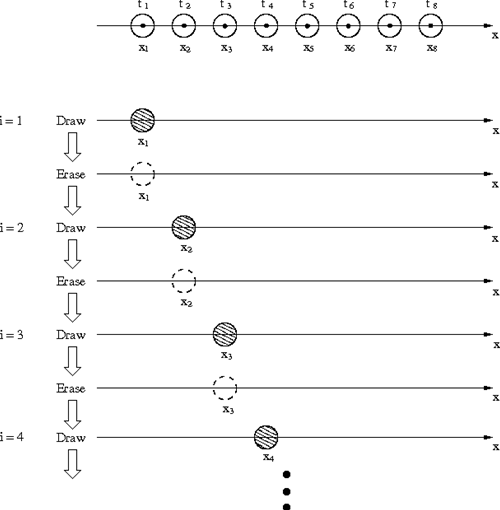Home > Resources > Making Animation with Scilab > 2. Basic idea of animation
Making Animation with Scilab

## 2. Basic idea of animation

First, we consider a simple one-dimensional case to illustrate the basic idea of animation.

Suppose a point moves on the x-axis with time. If the positions of the point are given for a fixed time interval as x = x1, x2, x3, ..., then an animation of the moving point can be made by looping the following 1-3. Assume the point is represented by a small circle. (See Figure 1.)

1. Erase the previous circle
2. Draw a circle at x = x i
3. i = i + 1Figure 1: Animation of a moving point (1D)